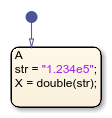# double

Convert string to double precision value

## Syntax

``X = double(str)``

## Description

example

````X = double(str)` converts the text in string `str` to a double-precision value. `str` contains text that represents a number. Text that represents a number can contain: DigitsA decimal pointA leading `+` or `-` signAn `e` preceding a power of 10 scale factor If `double` cannot convert the text to a number, it returns a `NaN` value.```

Note

Only Stateflow® charts that use MATLAB® as the action language support the `double` operator.

## Examples

expand all

Return a value of `-3.145`.

```h = "-3.145" x = double(h)```## Version History

Introduced in R2021b